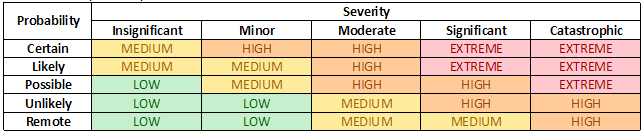A risk matrix is another of those tools in my toolbox that I’ll pull out on occasion to help my stakeholders determine the relative severity of project risks, and from there, the risk management strategy we want to pursue.

What is a risk matrix? Take it away, wikipedia:

Risk Matrix is used in the Risk Assessment process; it allows the severity of the risk of an event occurring to be determined.

risk is the total of each of the hazards that contribute to it. The risk of any particular hazard, H, can be defined as its probability, p, multiplied by its consequence, c. In layman’s terms: how likely it is to happen and how bad it would be if it happened.

Although there are complex mathematical formulas to derive risk severity and all kinds of risk matrix formats, I really like a simple one (see below) just to use as a visual, because it makes it much easier for me to explain of how severity and probability combine to make a risk more or less serious than if I tried to explain it with words alone.It’s a simple tool, and you won’t always need it, but if you think you might be able to put it to use, or would like to tweak to suit your needs, I’ve posted copies of the one above in Excel and Google Docs formats. Let me know if this comes in handy for you!